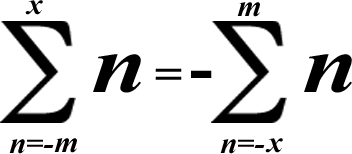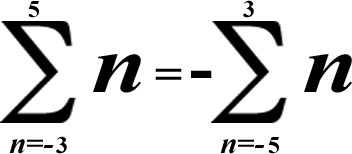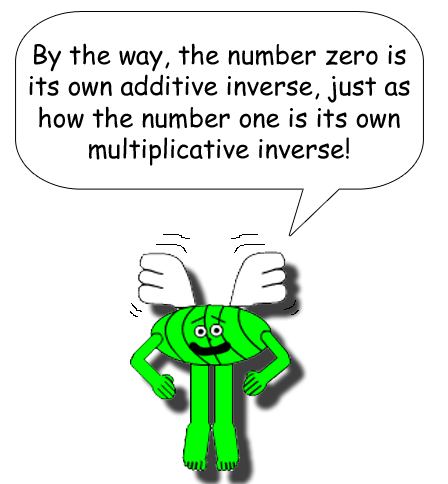# Super Summation 8(Of course the negative number always has to be below the sigma!) Notice how the variables m & x swap places. The variable below the sigma has to be multiplied by -1. You know that gives you a number's additive inverse, don't you?

## Example:(-3) + (-2) + (-1) + 0 + 1 + 2 + 3 + 4 + 5 = 4 + 5 = 9

[All of the additive inverses cancel each other out & you're left with 4 & 5 adding up to 9! Just below is the negative version of this summation!]

(-5) + (-4) + (-3) + (-2) + (-1) + 0 + 1 + 2 + 3 = (-5) + (-4) = -9

### The negative numbers are put in parentheses to prevent confusion with addition or subtraction.Here's Miss Zero Digit representing the number zero again! You may have already seen her in "Super Summation 2". This math trick is a sequel to it; plus, it's very similar to it!